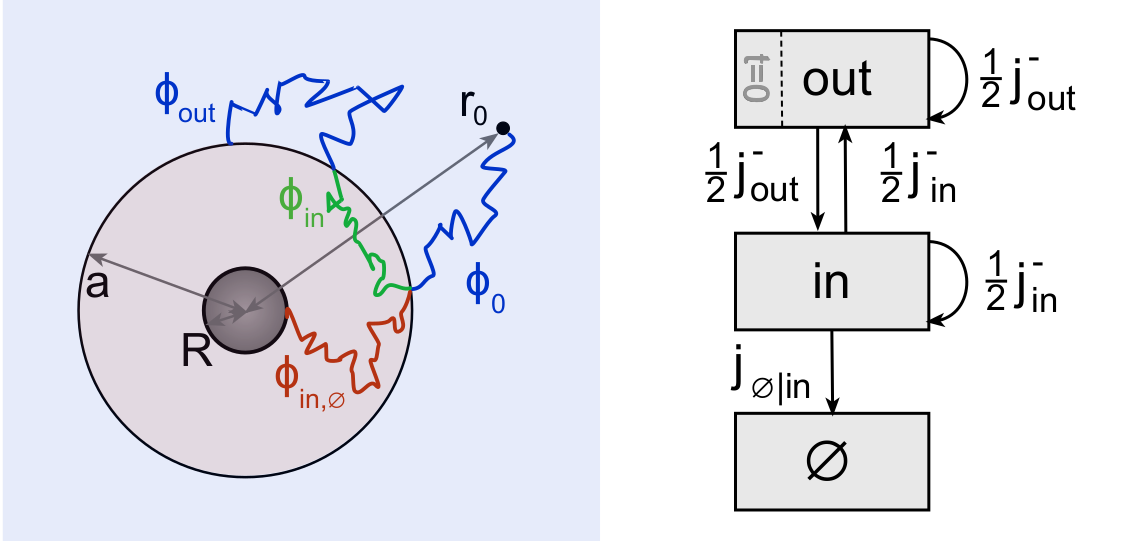Springe direkt zu Inhalt

Publication in the Journal of Physics AIn this example, the space around an absorbing target in the centre is partitioned into two domains. Dissecting the particle trajectories at the domain boundaries leads to a non-Markovian jump process with three states as depicted on the right.

First-passage problems in partitioned spaces can be solved by means of a novel generalised master equation.

News from Apr 30, 2021

Motivated by a range of biological applications related to the transport of molecules in cells, we present a modular framework to treat first-passage problems for diffusion in partitioned spaces. The spatial domains can differ with respect to their diffusivity, geometry, and dimensionality, but can also refer to transport modes alternating between diffusive, driven, or anomalous motion. The approach relies on a coarse-graining of the motion by dissecting the trajectories on domain boundaries or when the mode of transport changes, yielding a small set of states. The time evolution of the reduced model follows a generalized master equation (GME) for non-Markovian jump processes; the GME takes the form of a set of linear integro-differential equations in the occupation probabilities of the states and the corresponding probability fluxes. Further building blocks of the model are partial first-passage time (FPT) densities, which encode the transport behavior in each domain or state.

After an outline of the general framework for multiple domains, the approach is exemplified and validated for a target search problem with two domains in one- and three-dimensional space, first by exactly reproducing known results for an artificially divided, homogeneous space, and second by considering the situation of domains with distinct diffusivities. Analytical solutions for the FPT densities are given in Laplace domain and are complemented by numerical backtransforms yielding FPT densities over many decades in time, confirming that the geometry and heterogeneity of the space can introduce additional characteristic time scales.

Publication:

D. Frömberg and F. Höfling, Generalized master equation for first-passage problems in partitioned spaces, J. Phys. A: Math. Theor. 54, 215601 (2021).

3 / 3